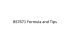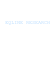# INF5481 RF Circuit, Theory and Design Assignment #3 Problem 1

## Transcription

INF5481 RF Circuit, Theory and Design Assignment #3 Problem 1
```INF5481 RF Circuit, Theory and Design
Assignment #3
Problem 1
We have the two port network in figure 1, which is a cascade of two identical
networks consisting of a single shunt and series impedance. Find the Z and
Y representation of the entire circuit.
ABCD1
ABCD1
ZB
ZB
ZA
ZA
ABCDseries
ABCDshunt
Figure 1: Diagram for problem 1
Problem 2
We have the circuit in figure 2, where we want to find the voltage over the
transformer and the transmission line; find VL .
ZG = 50 Ω
Z0 = 50 Ω
+
βl = 90◦
VL ZL = 25 Ω
−
1:2
VG = 3 V
Figure 2: Diagram for problem 2
1
INF5481: Assignment #3
Problem 3
Express the S parameters for a series resistance R = 100 Ω. The setup for
setting a2 = 0 is shown in figure 3.
ZG = Z0
Z0
R = 100 Ω
Z0
VG
ZL = Z0
S
Figure 3: Diagram for problem 3. Setup for finding the S-parameters for a
100 Ω resistor.
Problem 4
Repeat the previous problem, with the finite transmission lines connected to
a series inductor ZL = j10 Ω as shown in figure 4.
Z0 = 50 Ω
βl = 45◦
ZL = j10 Ω
L = 1.59 nH
Z0 = 50 Ω
βl = 45◦
S
Figure 4: Diagram for problem 4
Page 2 of 3
INF5481: Assignment #3
Problem 5
In the lab we measured on a short GCWP transmission line. The S parameters at 2.5 GHz where
0.00415 − 0.00331j
0.0949 − 0.933j
SGCPW =
0.0294 − 0.964j
−0.0470 + 0.0182j
(a)
Using the fact that S11 = Γ, calculate the VSWR and the input impedance
of the device. Using S21 , calculate the insertion loss in dB.
(b)
Since the line is relativly well matched to 50 Ω, S21 = e−γl . Ignoring the low
loss, calculate βl as the (negative) phase of S21 . Can the length l be uniquely
determined?
(c)
You have designed our own nifty gadget and is convinced it will save the
world. Before proceeding to fabrication, you would like to asses the performance when using the GCWP transmission lines to connect to the outside
world. You have the S parameters of your gadget (Sg ), describe how you
would find the total S parameters as depicted in figure 5.
goes here
?
SGCWP
Sg
SGCWP
Mandatory for INF9481 students: Insert your own circuit and carry
out the required calculations. Note: it does not have to save the world, nor
do you have to do it by hand.
Page 3 of 3
```

### Beyond classical chip design Exercise III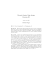### The Z-Brick® is a peice of test equipment to quickly check the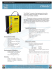### From the Desk of Dean G. De Vries Bock - ELCSA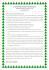### Vincent Camus### ÑÐ¾ÑÑÐ°Ð»ÑÐ½Ð¾-Ð³ÑÐ¼Ð°Ð½ÑÑÐ°ÑÐ½Ñ Ð°ÑÐ¿ÐµÐºÑÐ¸ ÑÐ¾Ð·Ð²Ð¸ÑÐºÑ ÑÑÑÐ°ÑÐ½Ð¾Ð³Ð¾ ÑÑÑÐ¿ÑÐ»ÑÑÑÐ²Ð°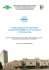### Why try Circuit?### Software Tools for Teaching High Frequency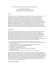### Meter Main with Load Center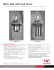### the brochure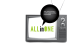### - K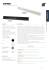### THE AUSTRALIAN NATIONAL UNIVERSITY Gerard Borg RADIOFREQUENCY ENGINEERING SAMPLE QUESTIONS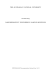### ac / dc electrical learning system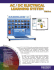### Homework #2 - Penn State Harrisburg Math/Computer Sciences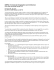### Success in the 16th Circuit, Jackson County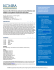### Motion Regarding Change of Domicile/Legal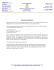### King Fahd University of Petroleum and Minerals College of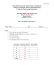### CIRCUITS WORKSHEET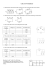### Supreme Court and the States: Mid-Term 2014-2015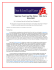### EET 1131 Lab #13 Multivibrators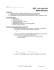### BS7671 Formula and Tips